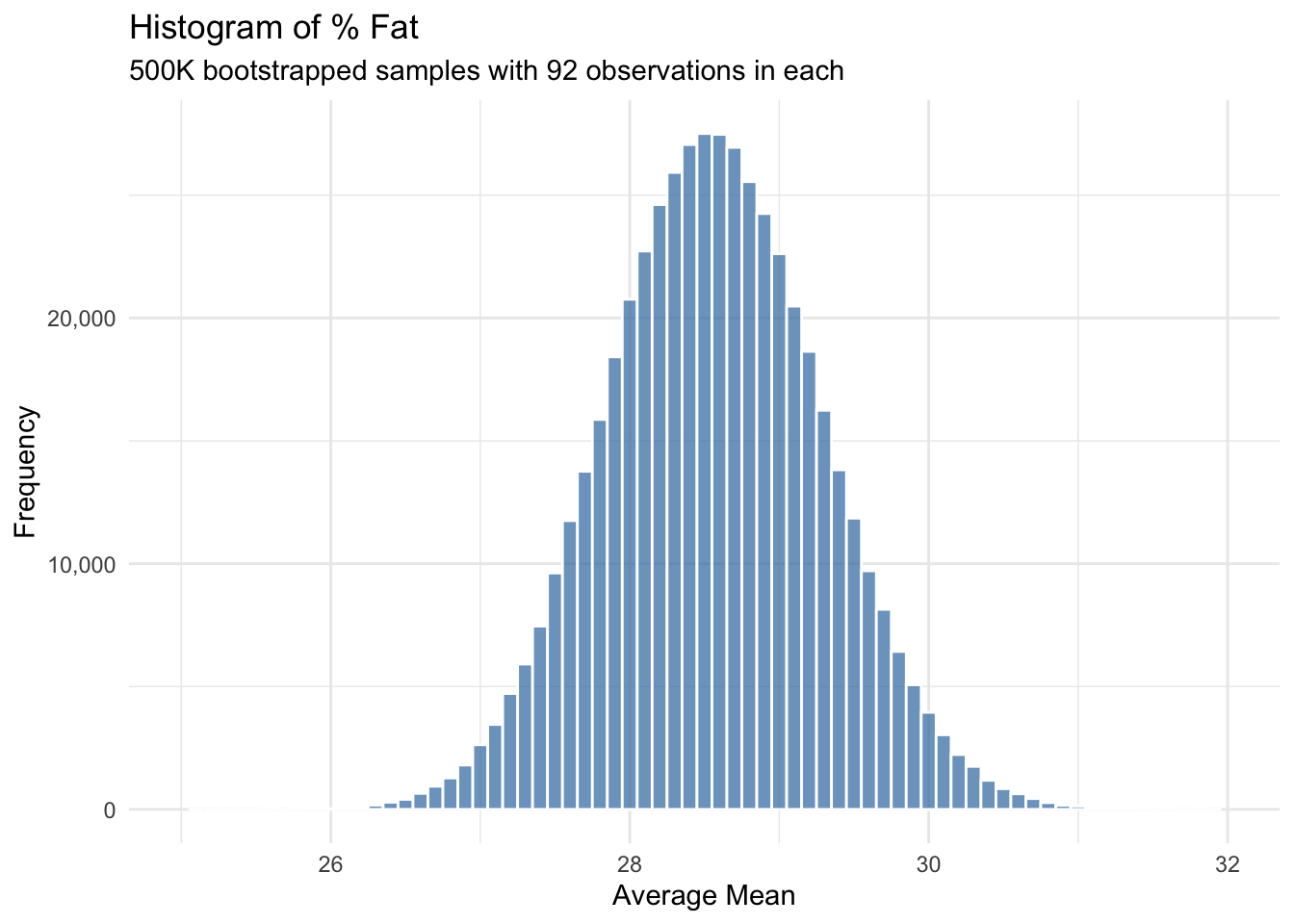Objective: recreate and visualize the 500K sampling distribtuion of means from this intro to bootstrapping in statistics post using R.

``````library(tidyverse)
library(rsample)``````

``df <- read_csv("https://statisticsbyjim.com/wp-content/uploads/2017/04/body_fat.csv")``

## Bootstrap resampling 500K

``````df_bs <- df %>%
bootstraps(times = 500000) %>%
mutate(average = map_dbl(splits, ~ mean(as.data.frame(.)\$`%Fat`))) ``````

## Visualize sampling distribution of means

``````df_bs %>%
ggplot(aes(average)) +
geom_histogram(binwidth = 0.1, alpha = 0.75,
color = 'white', fill = 'steelblue') +
scale_x_continuous(limits = c(25, 32)) +
scale_y_continuous(labels = scales::comma_format()) +
labs(title = "Histogram of % Fat",
subtitle = "500K bootstrapped samples with 92 observations in each",
x = "Average Mean", y = "Frequency") +
theme_minimal()``````